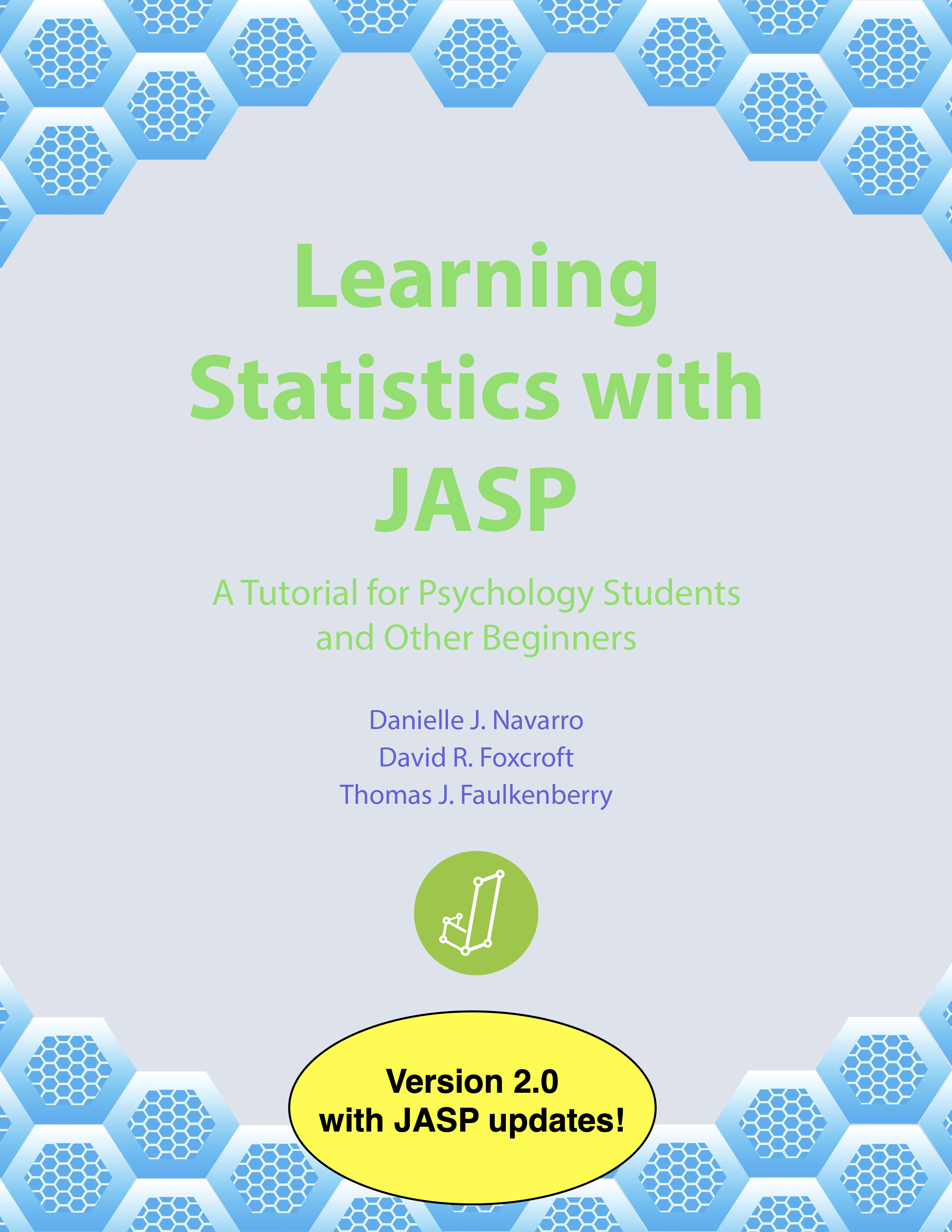## Learning Statistics with JASPWelcome to the homepage for the book Learning Statistics with JASP: A Tutorial for Psychology Students and Other Beginners, written by Danielle J. Navarro, David R. Foxcroft, and Thomas J. Faulkenberry.

Learning Statistics with JASP is a free textbook covering the basics of statistical inference for beginners in psychology and related applied disciplines. It uses the free software package JASP. Written in a lively, conversational style, it provides the reader with a perfect balance of readability and rigor, and gives students a modern view of statistical inference in the psychological and behavioral sciences.

• Complete book (pdf)
• Chapter 1: Why do we learn statistics? (pdf)
• Chapter 2: A brief introduction to research design (pdf)
• Chapter 3: Getting started with JASP (pdf)
• Chapter 4: Descriptive statistics (pdf)
• Chapter 5: Drawing graphs (pdf)
• Chapter 6: Introduction to probability (pdf)
• Chapter 7: Estimating unknown quantities from a sample (pdf)
• Chapter 8: Hypothesis testing (pdf)
• Chapter 9: Categorical data analysis (pdf)
• Chapter 10: Comparing two means (pdf)
• Chapter 11: Correlation and linear regression (pdf)
• Chapter 12: Comparing several means (one-way ANOVA) (pdf)
• Chapter 13: Factorial ANOVA (pdf)
• Chapter 14: Bayesian statistics (pdf)
• Epilogue (pdf)

### Data sets for book

The example datasets that are used in Learning Statistics with JASP are available for download in two ways.

• you can download one ZIP file containing all data files and save it to your computer (recommended)